# Maxwell Dusty Fluid Flow Past a Variable Thickness Porous Stretching Surface

Maxwell Dusty Fluid Flow Past a Variable Thickness Porous Stretching Surface
Basic and Applied Science Department, College of Engineering and Technology, Arab Academy for Science, Technology and Maritime Transport, Cairo P.O. BOX 2033, Egypt

Department of Physics & Engineering Mathematics, Faculty of Engineering-Mattaria, Helwan University, Cairo P.O. BOX 11718, Egypt

Corresponding Author Email:
moh_fathy79_6@aast.edu
Page:
1481-1489
|
DOI:
https://doi.org/10.18280/mmep.090606
21 September 2022
|
Accepted:
20 December 2022
|
Published:
31 December 2022
| Citation

OPEN ACCESS

Abstract:

An efficient Legendre-Galerkin method was applied to obtain a solution of the Maxwell dusty fluid flow past a changeable thickness stretching surface in porous medium. It is common to reduce a governing system of partial differential equations to a collection of ordinary differential equations using similarity transformation to obtain the asymptotic solutions using the Legendre-Galerkin technique. Comparison of the numerical results is made with previously published results under the special cases. The results are found to be in a good agreement. Numerical results were obtained for the skin-friction, velocity profiles of the fluid as well as velocity profiles of dusty particles for selected values of the governing parameters, such as velocity power index, wall thickness α, elasticity β, fluid particle interaction βv, porosity γ, and mass concentration

parameters. Also, the impacts of these parameter on the physical (surface heat flux and surface shear stress) and mechanical properties (surface cracking, strength, hardness, stiffness, etc.) are presented and investigated.
Keywords:

dusty fluid, maxwell fluid, stretching surface, Variable thickness, Legendre polynomials, Galerkin method

1. Introduction

Industrial applications like tap production, plastic and glass forming involve investigating flow across a stretching surface; some of these investigations focus on Newtonian fluids, while others look at non-Newtonian fluids. Many mathematical models simulate the non-Newtonian fluids in a computer simulation. Non-Newtonian fluid flow is more trustworthy from a technological standpoint than Newtonian fluid flow. For example, a viscoelastic non-Newtonian fluid can take on a variety of models, such as a third-grade model. A third-grade non-Newtonian fluid's shear thickening and shear thinning characteristics are illustrated in the study [1-3]. Hayat et al.  developed that in the effect of the chemical reaction. On the other hand, the impact of changeable viscosity due to temperature and thermal conductivity on the MHD flow past an extended cylinder by Salahuddin et al. . In the presence of a magnetic field, Radi and colleagues  studied the convective flow induced by an extended sheet.

Viscous and elastic procedures are offered by viscoelastic non-Newtonian fluid. The study [4-6] covers a number of these tactics. Using velocity slip, cross-diffusion effects were investigated by Baag et al. , Jayachandra Babu and Sandeep  presented the flow of MHD Williamson fluid through a stretched surface of varied thickness. Unsteadiness and micropolarity were studied by Elbashbeshy et al.  over a flat extendable surface.

The study [7-16] shows that the fluid molecule connection has an influence on the study of dusty fluid. Researchers Vajravelu and Nayfeh  applied the hydro attractive dusty fluid advancement across an extended surface and found that molecular connection, stacking, and pull all affected the stream features. Dusty fluid was studied by Gireesha et al.  as it traveled across an upwards expanding surface. Extending the review, Gireesha et al.  discussed the unsteady dusty fluid flow across a sheet in the vicinity of a nonhomogeneous heat source/sink. Thoughts of the thick distribution occurred to Ramesh et al.  when they were studying a stream of dusty fluid.

Using a nonhomogeneous heat source and sink, Gireesha et al.  introduced the dusty MHD flow of liquid across a tilted level expanding surface. An unstable dusty fluid heat transfer and flow across an exponentially stretched sheet exposed to suction were investigated numerically by Pavithra and Gireesha [12, 13]. In the occurrence of radiation impacts, Ramesh and Gireesha  introduced a dusty fluid flow across a stretched sheet. When heat generation/absorption and thermal radiation were present as well as an unstable mixed convective dusty fluid flow. Gireesha et al.  studied this flow. Using a stretched surface and heat production inside the porous medium, Rauta and Mishra  analyzed two stage flow.

Countless studies have looked at the effect of nanoparticles on the dusty fluid. For examples, Sandeep et al.  studied the radiative flow and heat transfer of a dusty nanofluid across an exponentially stretched sheet in the existence of a volume proportion of nano particles and dust. When it comes to MHD flow and heat transmission, Manjunatha and Gireesha  focused on the impacts of thermal conductivity and viscosity. MHD nanofluid with conducting dust particles embedded in it was researched by Naramgari and Sulochana  for its momentum and heat transfer behavior as it passed an extending sheet with the existence of a volume proportion of dust particles. MHD flow of nanofluid toward a nonlinear expandable surface with changing thickness was introduced by Daniel et al. . Bhatti et al.  showed how nonlinear thermal radiation affects the flow of a non-Newtonian dusty fluid with the inclusion of a solid substance past a porous channel. Utilizing the radiation effect, Reddy et al.  investigate the magneto-hydrodynamic boundary layer flow of Williamson nanofluid with heat and mass transfer across a stretched sheet with changing thickness. Powell-Eyring MHD nanofluid flow via non-directly extending surface of changeable thickness was presented by Hayat et al. . Elbashbeshy et al.  have studied a Maxwell dusty fluid flow across an extended sheet using the RKBS-45 method.

The current research aims to investigate Elbashbeshy et al.  in the present of the porosity medium effect but using the effective Legendre-Galerkin technique. Legendre-Galerkin technique is broadly utilized in numerous applications. As of late, numerous analysts applied it to take care of their issues. Cai and Qi  introduced the arrangement of general Volterra useful necessary conditions utilizing a completely discrete Legendre-Galerkin technique. Klahn et al.  presented the arrangement the completely nonlinear potential stream issue for water waves in a solitary even measurement. Schmutzhard  concentrated on the assessment of the prolate spheroidal wave capacities utilizing Legendre-Galerkin strategy. For weakly singular Fredholm integral equations of the second type and their accompanying eigenvalue issue, Panigrahi and Nelakanti  study Galerkin's technique utilizing Legendre polynomial basis functions. Fathy et al.  utilized Galerkin strategy with Legendre basis for tackling direct Fredholm integro-differential. Legendre–Galerkin was presented the arrangement of the biharmonic conditions by Zhuang and Chen . Sayed and Fathy  introduced how to obtain the flow of nanofluid through an upward cone.

2. Mathematical Modeling and Governing Equations

Consider a two-dimensional boundary layer flow of steady laminar Maxwell dusty fluid over a stretching surface. $a$ and $b$ are two constants in the equation that characterize the surface's profile: $y=a(x+b)^{(1-n) / 2}$ and $U_w=a(x+b)^n$. As shown in Figure 1(a), the mechanical cycle for this audit, in which the molten material is propelled from the pass-on to be stretched by a wrap-up wheel.

As seen in Figure 1 (b), the thickness of the expanding sheet gradually decreases until it reaches a uniform thickness. We assume that these constants are modest enough to keep the surface thin enough. Velocity index $n$ affects the thickness of a material's surface, which may either reduce $(n>1)$ or grow $(n<1)$ as the distance from the slit increases. As a side note, when $n=1$, the study is reduced to an essentially flat surface. Assumed in this study, the similarity variable and functions are valid if $n>-1$. In this case, $v_w=0$ indicates that the surface is completely impermeable. The stretching surface is selected along the $x$-direction of motion, and the $y$-direction is chosen normal to it. The following is a mathematical formulation for the model that governs this sort of flow [6, 14]:

$\frac{\partial u}{\partial x}+\frac{\partial v}{\partial y}=0$      (1)

$u \frac{\partial u}{\partial x}+v \frac{\partial u}{\partial y}+\lambda\left[u^2 \frac{\partial^2 u}{\partial x^2}+v^2 \frac{\partial^2 u}{\partial y^2}+2 u v\left(\frac{\partial^2 u}{\partial x \partial y}\right)\right]=v \frac{\partial^2 u}{\partial y^2}+\frac{K N}{\rho}\left(u_p-u\right)-\frac{v}{K^*} u,$      (2)

$\frac{\partial u_p}{\partial x}+\frac{\partial v_p}{\partial y}=0,$       (3)

$u_p \frac{\partial u_p}{\partial x}+v_p \frac{\partial u_p}{\partial y}=\frac{K N}{\rho}\left(u-u_p\right),$       (4)

subject to boundary conditions:

$u=U_w=a(x+b)^n, v=0$ at $y=a(x+b)^{(1-n) / 2},$      (5)

$u \rightarrow 0, v_p \rightarrow v, u_p \rightarrow 0$ as $y \rightarrow \infty,$

where, $u, v$ are two velocity components in each of the two orthogonal $x$ and $y$ directions of the fluid flow. $u_p$ and $v_p$ represent the $x$ - and $y$-velocity components of dust particles, respectively. The relaxation time parameter denotes by $\lambda$, while the dust particles number density and the Stokes resistance values symbolized by $N$ and $K$, respectively. When $\lambda>0$, the Maxwell dusty fluid is viscous and it is an inviscid fluid if $\lambda=0$. The fluid has a dynamic viscosity $v$ and a density $\rho . K^*=K_0(x+b)^{1-n}$ is the permeability of the porous media where $K_0$ is a constant. According to the study , as illustrated below, integrating dimensionless similarity variables simplifies the previous mathematical model as follow:

## 1.pngFigure 1. Physical model for the variable thickness stretching sheet

$\psi=\sqrt{\frac{n+1}{2} v a(x+b)^{n+1}} G(\xi), u=a(x+b)^n G^{\prime}(\xi),$

$v=-\sqrt{\frac{n+1}{2} v a(x+b)^{n-1}}\left(G(\xi)+\frac{n-1}{n+1} \xi G^{\prime}(\xi)\right),$

$\xi=\sqrt{\left(\frac{n+1}{2}\right) \frac{a(x+b)^{n-1}}{v}} y, u_p=a(x+b)^n H^{\prime}(\xi),$       (6)

$v_p=-\sqrt{\frac{n+1}{2} v a(x+b)^{n-1}}\left(H(\xi)+\frac{n-1}{n+1} \xi H^{\prime}(\xi)\right),$

where, $\xi$ is the similarity variable, $G(\xi)$ and $H(\xi)$ are dimensionless functions. The partial differential Eqns. (2)-(5) are changed into conventional differential conditions utilizing the likeness change procedure. The condition of coherence is fulfilled if a stream work $\psi$ is picked to such an extent that $u=\frac{\partial \psi}{\partial u}, v=-\frac{\partial \psi}{\partial x}$ which satisfies Eq. (1). After substituting Eqns. (6) into Eqns. (2)-(5), we get the following system of ordinary differential equations.

$G^{\prime \prime \prime}+G G^{\prime \prime}-\frac{2 n}{n+1} G^{'2}+\beta\left((3 n-1) G G^{\prime} G^{\prime \prime}-\frac{2 n(n-1)}{n+1} G^{'3}+\right.$

$\left.\frac{n-1}{2} \xi G^2 G^{\prime \prime}-\frac{n+1}{2} G^2 G^{\prime \prime \prime}\right)+\frac{2}{n+1} \ell \beta_v\left(H^{\prime}-G^{\prime}\right)-\gamma G^{\prime}=0,$     (7)

$n H^{\prime 2}-\frac{n+1}{2} H H^{\prime \prime}+\beta_v\left(H^{\prime}-G^{\prime}\right)=0$

depending on the following conditions:

$G(\alpha)=\alpha\left(\frac{1-n}{1+n}\right), G^{\prime}(\alpha)=1, \lim _{\xi \rightarrow \infty} G^{\prime}(\xi)=0,$

$\lim _{\xi \rightarrow \infty} H^{\prime}(\xi)=0, \lim _{\xi \rightarrow \infty} G(\xi)=\lim _{\xi \rightarrow \infty} H(\xi).$     (9)

The differentiation with regard to $\xi$ is denoted by primes. The wall thickness boundary, defined by the parameter $\alpha=a \sqrt{a(n+1) / 2 v}$, depicts the expanded surface's wall profile. The number of elasticity and the porosity parameter are computed in the forms $\beta=$ $\lambda a(x+b)^{n-1}$ and $\gamma=2 v / a K_0(n+1)$, respectively . If The mass of dusty particles denoted by $m$, the dusty particles mass concentration is computed by $\ell=N m / \rho$ and $\beta_v=$ $K / m a(x+b)^{n-1}$ is fluid particle interaction, where $m$ is the dusty particles mass. The similarity Eqns. (7) and (8), as well as the corresponding boundary conditions (9) become more obvious after using $G(\xi)=f(\xi-\alpha)=f(\eta)$ and $H(\xi)=F(\xi-\alpha)=F(\eta)$ is defined. They may be written as follows:

$f^{\prime \prime \prime}+f f^{\prime \prime}-\frac{2 n}{n+1} f^{\prime 2}+\beta\left((3 n-1) f f^{\prime} f^{\prime \prime}-\frac{2 n(n-1)}{n+1} f^{\prime 3}+\right.$

$\left.\frac{n-1}{2} \eta f^{\prime 2} f^{\prime \prime}-\frac{n+1}{2} f^2 f^{\prime \prime \prime}\right)+\frac{2}{n+1} \ell \beta_v\left(F^{\prime}-f^{\prime}\right)-\gamma f^{\prime}=0$,       (10)

$n F^{\prime 2}-\frac{n+1}{2} F F^{\prime \prime}+\beta_v\left(F^{\prime}-f^{\prime}\right)=0$,      (11)

depending on the following conditions:

$f(0)=\alpha\left(\frac{1-n}{1+n}\right), f^{\prime}(0)=1, \lim _{\eta \rightarrow \infty} f^{\prime}(\eta)=0,$      (12)

$\lim _{\eta \rightarrow \infty} F^{\prime}(\eta)=0, \lim _{\eta \rightarrow \infty} f(\eta)=\lim _{\eta \rightarrow \infty} F(\eta),$

and the differentiation with respect to $\eta$ is denoted by primes in this case. It's worth noting that the shape parameter $\mathrm{n}$ determines the boundary layer behavior, with $n=1$ reducing the boundary condition (12) to $f(0)=0$, which corresponds to an impermeable surface, and $n<1$ resulting in $f(0)>0$, which relates to suction. Furthermore, $n>1$ corresponds to $f(0)<0$, which denotes injection.

3. Legendre-Galerkin Method

The Legendre-Galerkin method is a strong and efficient numerical methodology for solving ordinary differential equations' linear and nonlinear boundary value issues. This numerical method's approach and primary definition have already been discussed in a number of publications. To make our computations easier, we should implement a suitable transformation to transfer the solution domain [0, ∞] to the domain [-1, 1]. Because the Legendre polynomials are in the computational interval [-1, 1], the transformation $\eta=\frac{\eta_{\infty}}{2}(\zeta+1)$ is employed to convert Eqns. (10)-(12) to the following forms in the first phase of this approach.

$\frac{8}{\eta_{\infty}^3} \frac{d^3 f}{d \zeta^3}+\frac{4}{\eta_{\infty}^2} f \frac{d^2 f}{d \zeta^2}-\frac{8 n}{\eta_{\infty}(n+1)}\left(\frac{d f}{d \zeta}\right)^2$$+\frac{8 \beta}{\eta_{\infty}^3}\left((3 n-1) f\left(\frac{d f}{d \zeta}\right)\left(\frac{d^2 f}{d \zeta^2}\right)-\frac{2 n(n-1)}{n+1}\left(\frac{d f}{d \zeta}\right)^3\right. \left.+\frac{n-1}{2}(\xi+1)\left(\frac{d f}{d \zeta}\right)^2\left(\frac{d^2 f}{d \zeta^2}\right)-\frac{n+1}{2} f^2\left(\frac{d^3 f}{d \zeta^3}\right)\right)$$+\frac{4}{n+1} \frac{\ell \beta_v}{\eta_{\infty}}\left(\frac{d F}{d \zeta}-\frac{d f}{d \zeta}\right)-\frac{2 \gamma}{\eta_{\infty}} \frac{d f}{d \zeta}=0,$       (13)

$\frac{4 n}{\eta_{\infty}^2}\left(\frac{d F}{d \zeta}\right)^2-\frac{2(n+1)}{\eta_{\infty}^2} F\left(\frac{d^2 f}{d \zeta^2}\right)+\frac{2 \beta_v}{\eta_{\infty}}\left(\frac{d F}{d \zeta}-\frac{d f}{d \zeta}\right)=0,$      (14)

with boundary conditions:

$f(-1)=\alpha\left(\frac{1-n}{1+n}\right), \frac{d f(-1)}{d \zeta}=\frac{\eta_{\infty}}{2}, \frac{d f(1)}{d \zeta}=\frac{d F(1)}{d \zeta}=0$, $f(1)=F(1)$,     (15)

Assume that the nonlinear system's unknowns in (13) and (14) have an approximate solution:

$f(\xi) \approx \sum_{i=0}^M c_j P_j(\zeta) \quad$ and $F(\xi) \approx \sum_{i=0}^M d_i P_i(\zeta).$        (16)

By inserting into the preceding system and using the Galerkin approach, the following results may be obtained.

$\left(\frac{n-1}{2}\right)(\xi+1) \times \sum_{j=0}^M \sum_{i=0}^M \sum_{k=0}^M c_j c_i c_k\left\langle\frac{d P_j(\zeta)}{d \zeta} \frac{d P_i(\zeta)}{d \zeta} \frac{d^3 P_k(\zeta)}{d \zeta^3}, P_r(\zeta)\right\rangle$

$+\frac{8 \beta}{\eta_{\infty}^3}\left(\sum_{j=0}^M c_j\left\langle\frac{d^3 P_j(\zeta)}{d \zeta^3}, P_r(\zeta)\right\rangle-\frac{2 n(n-1)}{n+1}\right.$

$\times \sum_{j=0}^M \sum_{i=0}^M \sum_{k=0}^M c_j c_i c_k\left\langle\frac{d P_j(\zeta)}{d \zeta} \frac{d P_i(\zeta)}{d \zeta} \frac{d P_k(\zeta)}{d \zeta}, P_r(\zeta)\right\rangle+(3 n-1)$

$\times \sum_{j=0}^M \sum_{i=0}^M \sum_{k=0}^M c_j c_i c_k\left\langle\frac{d P_j(\zeta)}{d \zeta} \frac{d P_i(\zeta)}{d \zeta} \frac{d^2 P_k(\zeta)}{d \zeta^2}, P_r(\zeta)\right\rangle+\frac{4 \ell \beta_v}{\eta_{\infty}^3(n+1)} \sum_{j=0}^M d_j\left\langle\frac{d P_j(\zeta)}{d \zeta}, P_r(\zeta)\right\rangle$

$+\frac{4}{\eta_{\infty}^2} \sum_{j=0}^M \sum_{i=0}^M c_j c_i\left\langle\frac{d P_j(\zeta)}{d \zeta} \frac{d^2 P_i(\zeta)}{d \zeta^2}, P_r(\zeta)\right\rangle-\left(\frac{n+1}{2}\right)$

$\left.\times \sum_{j=0}^M \sum_{i=0}^M \sum_{k=0}^M c_j c_i c_k\left\langle\frac{d P_j(\zeta)}{d \zeta} \frac{d P_i(\zeta)}{d \zeta} \frac{d^3 P_k(\zeta)}{d \zeta^3}, P_r(\zeta)\right\rangle\right)$

$-\frac{8 n}{\eta_{\infty}(n+1)} \sum_{j=0}^M \sum_{i=0}^M c_j c_i\left\langle\frac{d P_j(\zeta)}{d \zeta} \frac{d P_i(\zeta)}{d \zeta}, P_r(\zeta)\right\rangle$

$-\frac{1}{\eta_{\infty}}\left(\frac{4 \ell \beta_v}{n+1}+2 \gamma\right) \sum_{j=0}^M c_j\left\langle\frac{d P_j(\zeta)}{d \zeta}, P_r(\zeta)\right\rangle=0,$        (17)

\begin{aligned} \frac{4 n}{\eta_{\infty}^2} \sum_{j=0}^M \sum_{i=0}^M d_j d_i\left\langle\frac{d P_j(\zeta)}{d \zeta}\right. & \left.\frac{d P_i(\zeta)}{d \zeta}, P_r(\zeta)\right\rangle-\frac{2(n+1)}{\eta_{\infty}^2} \sum_{j=0}^M \sum_{i=0}^M d_j d_i\left\langle\frac{d P_j(\zeta)}{d \zeta} \frac{d^2 P_i(\zeta)}{d \zeta^2}, P_r(\zeta)\right\rangle \\ & +\frac{2 \beta_v}{\eta_{\infty}} \sum_{j=0}^M\left(d_j-c_j\right)\left\langle\frac{d P_j(\zeta)}{d \zeta}, P_r(\zeta)\right\rangle=0\end{aligned}     (18)

in terms of the boundaries:

$\sum_{j=0}^M(-1)^j c_j=\alpha\left(\frac{1-n}{1+n}\right), \sum_{j=0}^M(-1)^{j+1} j(j+1) c_j=\eta_{\infty},$

$\sum_{j=0}^M j(j+1) c_j=0, \sum_{j=0}^M j(j+1) d_j=0, \sum_{j=0}^M\left(c_j-d_j\right)=0,$     (19)

where, 〈⋅,⋅〉 is defined as the inner product:

$\langle\varphi(\zeta), \omega(\zeta)\rangle=\int_{-1}^1 \varphi(\zeta) \omega(\zeta) d \zeta$

The matrix form of the nonlinear system (17) and (18) with condition (19) is:

$\mathbf{H} \mathbf{c}+\boldsymbol{\Psi} \tilde{\mathbf{c}}+\boldsymbol{\Theta} \overline{\mathbf{c}}=\mathbf{b}$     (20)

where,

$\mathbf{H}=\left(\begin{array}{ll}\mathbf{H}_{f_1} & \mathbf{H}_{F_1} \\ \mathbf{H}_{f_2} & \mathbf{H}_{F_2}\end{array}\right), \boldsymbol{\Psi}=\left(\begin{array}{cc}\boldsymbol{\Psi}_{f_1} & \boldsymbol{O} \\ \boldsymbol{O} & \boldsymbol{\Psi}_{F_2}\end{array}\right), \boldsymbol{\Theta}=\left(\begin{array}{c}\boldsymbol{\Theta}_{f_1} \\ \boldsymbol{O}\end{array}\right), \mathbf{b}=\left(\begin{array}{l}\mathbf{b}_1 \\ \mathbf{b}_2\end{array}\right),$

and

{{\mathbf{H}}_{{{\mathbf{f}}_{\mathbf{1}}}}}=\left( \begin{align} & \frac{{{\vartheta }_{j,r}}}{\begin{align} & {{(-1)}^{j}} \\ & {{(-1)}^{j+1}}j(j+1) \\ & j(j+1) \\\end{align}} \\ & \\\end{align} \right){{\mathbf{H}}_{{{\mathbf{f}}_{\mathbf{2}}}}}=\left( \begin{matrix} \frac{{{\omega }_{j,r}}}{0} \\ 0 \\\end{matrix} \right)

${{\mathbf{H}}_{{{\mathbf{F}}_{\mathbf{1}}}}}=\left( \begin{matrix} \frac{{{\chi }_{j,r}}}{0} \\ 0 \\ 0 \\\end{matrix} \right){{\mathbf{H}}_{{{F}_{2}}}}=\left( \begin{matrix} \frac{{{\zeta }_{j,r}}}{j(j+1)} \\ 1 \\\end{matrix} \right)$

$c=\operatorname{span}\left\{c_j\right\}, \tilde{c}=\left\{\operatorname{span}\left\{c_i c_j\right\}, \operatorname{span}\left\{d_i d_j\right\}\right\},$

$\bar{c}=\left\{\operatorname{span}\left\{c_i c_j c_k\right\}, \operatorname{span}\left\{d_i d_j d_k\right\}\right\},$

$\left.b_1=\left(\begin{array}{llllllll}0 & 0 & 0 & 0 & 0 & 0 & \cdots & \alpha\left(\frac{1-n}{1+n}\right.\end{array}\right) \quad \eta_{\infty} \quad 0\right)^T,$

$b_2=\left(\begin{array}{llllllllll}0 & 0 & 0 & 0 & 0 & 0 & \cdots & 0 & 0 & 0\end{array}\right)^T,$

$\vartheta_{j, r}=\frac{8}{\eta_{\infty}^3}\left\langle\frac{d^3 P_j(\zeta)}{d \zeta^3}, P_r(\zeta)\right\rangle$$-\left(\frac{4}{n+1} \frac{\ell \beta_v}{\eta_{\infty}}+\frac{2 \gamma}{\eta_{\infty}}\right)\left\langle\frac{d P_j(\zeta)}{d \zeta}, P_r(\zeta)\right\rangle, (21) \omega_{j, r}=-\frac{2 \beta_v}{\eta_{\infty}}\left\langle\frac{d P_j(\zeta)}{d \zeta}, P_r(\zeta)\right\rangle, (22) \chi_{j, r}=\frac{4}{n+1} \frac{\ell \beta_v}{\eta_{\infty}}\left\langle\frac{d P_j(\zeta)}{d \zeta}, P_r(\zeta)\right\rangle, (23) \varsigma_{j, r}=\frac{2 \beta_v}{\eta_{\infty}}\left\langle\frac{d P_j(\zeta)}{d \zeta}, P_r(\zeta)\right\rangle, (24) \boldsymbol{\Psi}_{f_1}=\left\{\Lambda_{i, j, r}\right\}=-\frac{8 n}{\eta_{\infty}(n+1)}\left\langle\frac{d P_j(\zeta)}{d \zeta} \frac{d P_i(\zeta)}{d \zeta}, P_r(\zeta)\right\rangle$$+\frac{4}{\eta_{\infty}^2}\left\langle P_j(\zeta) \frac{d^2 P_i(\zeta)}{d \zeta^2}, P_r(\zeta)\right\rangle$      (25)

\begin{aligned} \boldsymbol{\Psi}_{\boldsymbol{F}_1}= & \left\{\kappa_{i, j, k, r}\right\}=\frac{4 n}{\eta_{\infty}^2}\left\langle\frac{d P_j(\zeta)}{d \zeta} \frac{d P_i(\zeta)}{d \zeta}, P_r(\zeta)\right\rangle \\ & -\frac{2(n+1)}{\eta_{\infty}^2}\left\langle P_j(\zeta) \frac{d^2 P_i(\zeta)}{d \zeta^2}, P_r(\zeta)\right\rangle,\end{aligned}

$\boldsymbol{\Psi}_{\boldsymbol{F}_{\mathbf{1}}}=\left\{\kappa_{i, j, k, r}\right\}=\frac{4 n}{\eta_{\infty}^2}\left\langle\frac{d P_j(\zeta)}{d \zeta} \frac{d P_i(\zeta)}{d \zeta}, P_r(\zeta)\right\rangle$$-\frac{2(n+1)}{\eta_{\infty}^2}\left\langle P_j(\zeta) \frac{d^2 P_i(\zeta)}{d \zeta^2}, P_r(\zeta)\right\rangle, (26) \boldsymbol{\Theta}_{f_1}=\left\{\Omega_{i, j, k, r}\right\}$$=\left(\frac{n-1}{2}\right)(\xi+1)\left\langle\frac{d P_j(\zeta)}{d \zeta} \frac{d P_i(\zeta)}{d \zeta} \frac{d^2 P_k(\zeta)}{d \zeta^2}, P_r(\zeta)\right\rangle$$-\frac{2 n(n-1)}{n+1}\left\langle\frac{d P_j(\zeta)}{d \zeta} \frac{d P_i(\zeta)}{d \zeta} \frac{d P_k(\zeta)}{d \zeta}, P_r(\zeta), P_r(\xi)\right\rangle +\frac{8 \beta}{\eta_{\infty}^3}\left((3 n-1)\left\langle P_j(\zeta) \frac{d P_i(\zeta)}{d \zeta} \frac{d^3 P_k(\zeta)}{d \zeta^3}, P_r(\zeta)\right\rangle-\frac{n+1}{2}\left\langle P_j(\zeta) P_i(\zeta) \frac{d^3 P_k(\zeta)}{d \zeta^3}, P_r(\zeta)\right\rangle\right). (27) The preceding terms (21)-(27) are evaluated using theorems was discussed [34-37] by Fathy. Newton's iteration method used to obtain the solution of the nonlinear Eq. (20) of 3 M+3 algebraic equations with 3 M+3 unknowns c_j and d_j. Solving the system of equations and substituting c_j and d_j in Eq. (16) may yield the asymptotic solutions. We need to use the inverse transformation \zeta=\frac{2}{\eta_{\infty}} \eta-1 in order to solve our problem. After that, we've identified an approximate solution to our problem. 4. Results Using the Legendre-Galerkin method, the semi-analytic solution for the couple system (13)-(15) is achieved. According to the issue parameters, possible semi-analytic solutions may be found in Table 1 for M=10. The fluid f^{\prime}(\eta) and the dusty particle F^{\prime}(\eta) velocity profiles are influenced by a variety of parameters. C_f is an essential physical quantity in our investigation since it is expressed as; C_f=-2 \sqrt{\frac{(n+1)}{2}}\left(\operatorname{Re}_x\right)^{-\frac{1}{2}} f^{\prime \prime}(0) (28) where, C_f \sqrt{\operatorname{Re}_x}=-2 \sqrt{(n+1) / 2} f^{\prime \prime}(0) is the modified skin-friction and \operatorname{Re}_x=U_w(x+ b) / v is the local Reynold number . Using numerical data provided by [24, 38, 39]. We were able to verify the precision of our technique. It is clear from Table 2 and Table 3 that numerical values for -f^{\prime \prime}(0) at various n values (ignoring the influence of the porous medium) are in great agreement [24, 38, 39]. There are three types of flow: laminar, transient, and turbulent. The ratio of inertia to viscosity is determined by a dimensionless number known as the Reynold number. Friction between a stretched surface and adjacent fluid particles, known as "skin friction," creates a drag force whose magnitude depends on the Reynolds number. There are two kinds of friction: local skin friction and modified skin friction, which take into consideration the wall profile's influence on the Reynold number. According to the data in Table 4 , the following parameters have an impact on the modified skin friction: velocity power index n, wall thickness \alpha, elasticity \beta, fluid particle interaction \beta_v, porosity \gamma, and mass concentration \ell parameters. Table 1. Some semi-analytic solutions obtained for M=10  Parameters Solutions n=2, \beta=0.2, \alpha=0.25, \beta_v=0.4, \gamma=0.2, \ell=0.3 f(\eta)=-0.0833+\eta-0.6458 \eta^2+0.2783 \eta^3 -0.08165 \eta^4+0.01489 \eta^5-0.00123 \eta^6 -0.000074 \eta^7+0.000027 \eta^8 +2.7 \times 10^{-7} \eta^9+7.81 \times 10^{-8} \eta^{10}. F(\eta)=0.5368+0.22008 \eta-0.13369 \eta^2 +0.0554 \eta^3-0.01699 \eta^4+0.00377 \eta^5 -0.0005664 \eta^6+0.000051 \eta^7 -0.00000204 \eta^8-2.92 \times 10^{-8} \eta^9$$+3.82 \times 10^{-9} \eta^{10}$. $n=0.5$, $\beta=0.2$, $\alpha=1.2$, $\beta_v=1$, $\gamma=0.2$, $\ell=0.6$ $f(\eta)=0.4+\eta-0.7385 \eta^2+0.3208 \eta^3$ $-0.0826 \eta^4+0.01 \eta^5+0.00061 \eta^6$ $-0.000418 \eta^7+0.0000637 \eta^8$ $-0.0000045 \eta^9+1.26 \times 10^{-7} \eta^{10}$. $F(\eta)=0.7479+0.469 \eta-0.3747 \eta^2$ $+0.189 \eta^3-0.06483 \eta^4$$+0.0152 \eta^5-0.00236 \eta^6$ $+0.00022 \eta^7-0.000011 \eta^8$ $+1.79 \times 10^{-7} \eta^9+5.35 \times 10^{-9} \eta^{10}$.

Table 2. Comparing the value of $-f^{\prime \prime}(0)$ to that of other technique at various α=0.25

 n α=0.25 Results  Results  Results  Present work 10 1.14332 1.1433 1.1433 1.14332 9 1.14039 1.1404 1.1404 1.14039 7 1.13228 1.1323 1.1322 1.13228 5 1.11859 1.1186 1.1186 1.11859 3 1.09049 1.0905 1.0904 1.09049 1 1.00000 1.0000 1.0000 1.00000 0.5 0.93382 0.9338 0.9337 0.93382 0 0.78427 0.7844 0.7843 0.78427 -1/3 0.50000 0.5000 0.5000 0.50000 -0.5 0.08333 0.0833 0.0832 0.08310

4.1 Velocity power index

According to the first part of Table $3, C_f$ rises with the increasing of velocity power index. Physically, the frictional impact of surface-to-fluid contact may be increased by raising power index $n$.

4.2 Wall thickness parameter

The modified-skin friction increases for n<1 and decreases for n>1 under the effect of the wall thickness parameter, as shown in Table 3 and Table 4 second and third sections. When the suction process is present, one may state that the mechanical characteristics of the surface (hardness, strength, etc.) can be enhanced.

4.3 Elasticity parameter

When the β is high, the viscosity and resistivity of the fluid rise, causing greater friction within the fluid and lowering the flow velocity. As seen in the fourth and fifth halves of Table 3 and Table 4, these consequences manifest themselves in an increase in modified-skin friction with rising β, the modified skin friction increases higher for n>1 than for n<1.

Table 3. Comparing the value of $-f^{\prime \prime}(0)$ to that of other technique at various α=0.5

 n α=0.5 Results  Results  Results  Present work 10 1.06032 1.0603 1.0603 1.06032 9 1.05891 1.0589 1.0588 1.05891 7 1.05504 1.0550 1.0551 1.05504 5 1.04861 1.0486 1.0486 1.04861 3 1.03586 1.0359 1.0358 1.03586 1 1.00000 1.0000 1.0000 1.00000 0.5 0.97994 0.9799 0.9798 0.97994 0 0.95764 0.9576 0.9577 0.95764 -1/3 1.00000 1.0000 1.0000 1.00000 -0.5 1.16682 1.1667 1.6666 1.16682

Table 4. The values of $-f^{\prime \prime}(0)$ and modified skin friction for variation of some parameters

 $n$ $\alpha$ $\boldsymbol{\beta}$ $\boldsymbol{\beta}_v$ $\gamma$ $\ell$ $-\boldsymbol{f}^{\prime \prime}(\mathbf{0})$ $C_f \sqrt{R e_x}$ n 0 0.25 0.2 0.4 0.2 0.3 0.964870 1.364532 0.5 0.25 0.2 0.4 0.2 0.3 1.098202 1.902142 2 0.25 0.2 0.4 0.2 0.3 1.289897 3.159589 $\underset{n<1} {\alpha}$ 0.5 0.1 0.2 0.4 0.2 0.3 1.064924 1.844504 0.5 0.8 0.2 0.4 0.2 0.3 1.232053 2.133979 0.5 1.2 0.2 0.4 0.2 0.3 1.342970 2.326093 $\stackrel{\alpha} {n>1}$ 1.2 0.1 0.2 0.4 0.2 0.3 1.215377 2.549397 1.2 0.8 0.2 0.4 0.2 0.3 1.168720 2.451527 1.2 1.2 0.2 0.4 0.2 0.3 1.143230 2.398060 $\beta$ $n<1$ 0.5 0.25 0 0.4 0.2 0.3 1.089756 1.887513 0.5 0.25 0.3 0.4 0.2 0.3 1.098202 1.902142 0.5 0.25 0.4 0.4 0.2 0.3 1.107398 1.918071 $\beta$ $n>1$ 1.2 0.25 0 0.4 0.2 0.3 1.145629 2.403093 1.2 0.25 0.3 0.4 0.2 0.3 1.205151 2.527946 1.2 0.25 0.4 0.4 0.2 0.3 1.261721 2.646608 $\beta_v$ $n>1$ 0.5 0.25 0.2 0.2 0.2 0.3 1.077985 1.867126 0.5 0.25 0.2 0.5 0.2 0.3 1.105757 1.915227 0.5 0.25 0.2 1.0 0.2 0.3 1.129529 1.956403 $\beta_v$ $n<1$ 1.2 0.25 0.2 0.2 0.2 0.3 1.191339 2.498974 1.2 0.25 0.2 0.5 0.2 0.3 1.210719 2.539626 1.2 0.25 0.2 1.0 0.2 0.3 1.230486 2.581091 $\gamma$ $n<1$ 0.5 0.25 0.2 0.4 0.0 0.3 1.000613 1.733112 0.5 0.25 0.2 0.4 0.2 0.3 1.098202 1.902142 0.5 0.25 0.2 0.4 0.4 0.3 1.188148 2.057933 $\gamma$ $n>1$ 1.2 0.25 0.2 0.4 0.0 0.3 1.120629 2.350651 1.2 0.25 0.2 0.4 0.2 0.3 1.205151 2.527946 1.2 0.25 0.2 0.4 0.4 0.3 1.284265 2.693898 $\ell$ $n<1$ 0.5 0.25 0.2 0.4 0.2 0.0 1.046844 1.813188 0.5 0.25 0.2 0.4 0.2 0.3 1.098202 1.902142 0.5 0.25 0.2 0.4 0.2 0.6 1.147365 1.987295 $\ell$ $n>1$ 1.2 0.25 0.2 0.4 0.2 0.0 1.172187 2.458800 1.2 0.25 0.2 0.4 0.2 0.3 1.205151 2.527946 1.2 0.25 0.2 0.4 0.2 0.6 1.237239 2.595255

4.4 Fluid particle interaction parameter

From the sixth and seventh part of Table 3 and Table 4 we note that modified-skin friction increases with increasing the fluid particle interaction parameter $\beta_v$.

4.5 Porosity parameter

An increasing in porosity parameter $\gamma$ leads to an increasing in modified-skin friction as seen in the eighth and tenth halves of Table 3 and Table 4.

4.6 Mass concentration of dusty particles

We note that modified-skin friction increases as mass concentration of dusty particles $\ell$ increases, As seen in the last two parts of Table 3 and Table 4 .

5. Discussions

The stretching surface profile (see Figure 1b) and stretching wall velocity are both defined by the index power $n$. The surface becomes thinner as the value of $n$ increases. The index power $n$ is also used to define the stretched wall velocity $U_w$. The parameter's impact on dimensionless velocity may be seen in Figure 2. By increasing the velocity index power $n$, the stretching surface velocity and the profile surface curvature is raised. As can be seen in Figure $2 \mathrm{~b}$, the momentum boundary layer gets thinner as the dimensionless velocity drops. This effect is reversed when $\eta>0.85$. Dusty particles' velocity drops in the same proportion. As a consequence, the amount of resistive force needed to keep the Maxwell dusty fluid from forming grows as this value is increased. For Newtonian dusty fluid, when the index power grows, the flow velocity decreases until $\eta=1.5$ then it rises with increasing the value of $n$, but the particle velocity falls as shown in Figure 2a.

## 2.png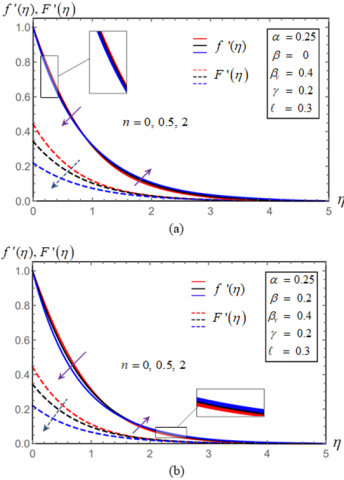Figure 2. (a) Effect of n for Newtonian dusty fluid and (b) Effect of n for Maxwell dusty fluid

Figure 3 discusses the results of an investigation into the relationship between the fluid flow velocity and the wall thickness parameter $\alpha$. As seen in Figure 3a, the boundary layer gets thinner and thinner as the velocity falls when $\alpha$ increases for $n<1$, but Figure 3b demonstrates that the boundary layer thickens as the velocity rises for $n>1$. Velocity of dusty particles has the similar pattern.

## 3.png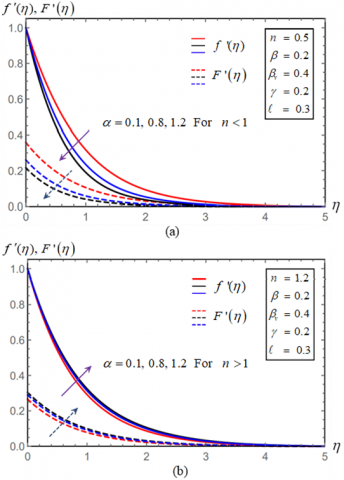Figure 3. (a) Effect of α  for n<1 and (b) Effect of α for n>1

The elasticity parameter of the Deborah number is a dimensionless number that reflects the ratio of time scale flow to relaxation time flow. When determining the type of unstable flow, the time scale is used. A viscous fluid, for example, requires a certain amount of time to relax once shear tension has ceased to exert pressure on it. It can be shown in Figure 4 that increasing the elasticity parameter decreases the dimensionless velocity. This is due to the fact that increasing the viscosity of a fluid increases the fluid's resistance. Figure 4b shows that for n>1, the elasticity parameter has a somewhat larger effect than for n<1 (Figure 4a). Additionally, when the elasticity value is raised, dust particle velocity falls slightly.

## 4.png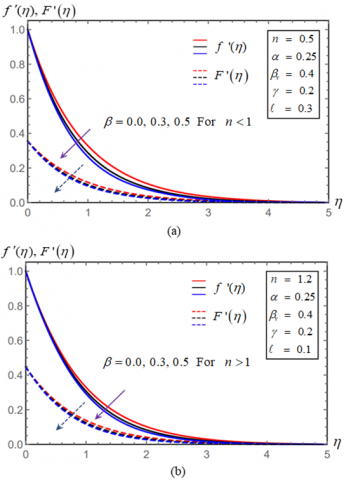Figure 4. (a) Effect of β for n<1 and (b) Effect of β for n>1

The dust phase's velocity rises while the fluid phase's velocity decreases as the value of $\beta_v$ increases in Figure 5. Because of the considerable contact between the fluid and particle phases, the particle phase may impose an opposing force on the fluid phase until the particle velocity is equal to the fluid velocity, allowing this effect to occur.

## 5.png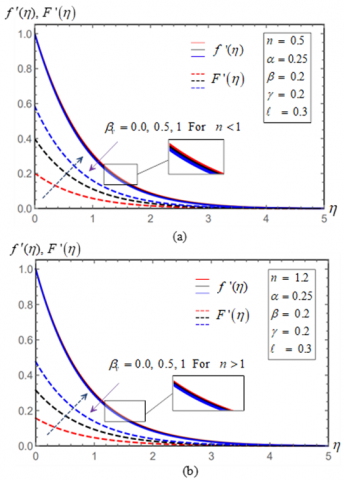Figure 5. (a) Effect of $\beta_v$ for n<1 and (b) Effect of $\beta_v$ for n>1

Figures 6 demonstrate the impact of the porosity parameter on velocity profiles in the fluid and dust phases. Increasing the porosity parameter decreases the velocity profiles for both the fluid and dust phases in the figure. To explain this, raising the porosity parameter causes the pores in the porous material to enlarge, resulting in a decrease in velocity profiles.

## 6.png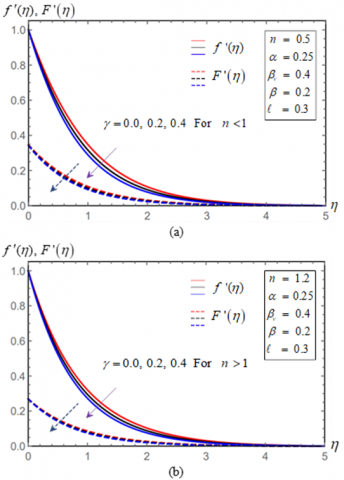Figure 6. (a) Effect of γ for n<1 and (b) Effect of γ for n>1

It is seen in Figure 7 that mass concentration of dust particles $\ell$ affects both the fluid and dust velocity profiles. The figure illustrates how increasing $\ell$ reduces the fluid and particle phase velocity profiles. As the mass concentration of dust particles rises, the velocity boundary layers are noticeably diminished.

## 7.png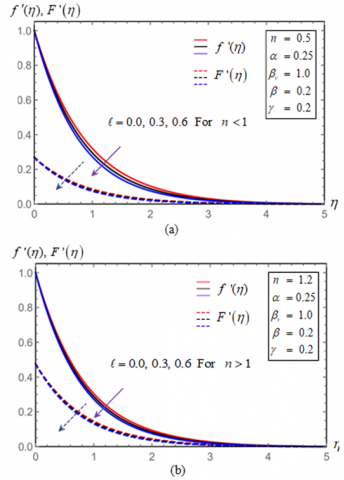Figure 7. (a) Effect of $\ell$ for n<1 and (b) Effect of $\ell$ for n>1

6. Conclusions

Maxwell dusty fluid on a stretched surface with varying thickness is studied numerically. A similarity transformation for the governing boundary layer equations was done based on the velocity power index, wall thickness, elasticity, fluid-particle interaction, porosity, and dust particle mass concentration variables. The Legendre-Galerkin algorithm is used for obtaining the flow and dust velocities. Flow velocity has been shown to be affected by the values of these factors. The results of the modified-skin friction tests have been compiled. A brief summary of the study's results is provided below.

• Rise in velocity power index, elasticity, fluid particle interaction, mass concentration of dust particles, and porosity characteristics all contribute to a progressive increase in friction factor.
• Skin-friction modification rises as the wall thickness parameter α (for n<1) increases. However, for n>1, it decreases in value as α raises.
• When n is increased, the velocity in the boundary layer falls near the surface and then increases again.
• The fluid and dusty particles' velocity is reduced when the elasticity β, porosity γ, and mass concentration of dusty particles $\ell$ are increased.
• Increasing the fluid-particle interaction parameter βv reduces the fluid phase's velocity and increases the particle phase's velocity.

Increases in the wall thickness parameter α has a negative impact on fluid phase velocity and particle phase velocity for n<1 and has a positive effect on n>1.

References

 Hayat, T., Khan, M.I., Waqas, M., Alsaedi, A., Yasmeen, T. (2017). Diffusion of chemically reactive species in third grade fluid flow over an exponentially stretching sheet considering magnetic field effects. Chinese Journal of Chemical Engineering, 25(3): 257-263. https://doi.org/10.1016/j.cjche.2016.06.008

 Salahuddin, T., Malik, M.Y., Hussain, A., Bilal, S., Awais, M. (2015). Effects of transverse magnetic field with variable thermal conductivity on tangent hyperbolic fluid with exponentially varying viscosity. AIP Advances, 5(12): 127103. https://doi.org/10.1063/1.4937366

 Rashidi, M.M., Bagheri, S., Momoniat, E., Freidoonimehr, N. (2017). Entropy analysis of convective MHD flow of third grade non-newtonian fluid over a stretching sheet. Ain Shams Engineering Journal, 8(1): 77-85. https://doi.org/10.1016/j.asej.2015.08.012

 Baag, S., Mishra, S.R., Dash, G.C., Acharya, M.R. (2017). Entropy generation analysis for viscoelastic MHD flow over a stretching sheet embedded in a porous medium. Ain Shams Engineering Journal, 8(4): 623-632. https://doi.org/10.1016/j.asej.2015.10.017

 Jayachandra Babu, M., Sandeep, N. (2016). MHD non-newtonian fluid flow over a slendering stretching sheet in the presence of cross-diffusion effects. Alexandria Engineering Journal, 55(3): 2193-2201. https://doi.org/10.1016/j.aej.2016.06.009

 Elbashbeshya, E.M.A., Abdelgaber, K.M., Asker, H.G. (2018). Unsteady flow of micropolar maxwell fluid over stretching surface. Journal of the Egyptian Mathematical Society, 26(2): 245-258. https://doi.org/10.21608/joems.2018.2576.1011

 Vajravelu, K., Nayfeh, J. (1992). Hydromagnetic flow of a dusty fluid over a stretching sheet. International Journal of Non-Linear Mechanics, 27(6): 937-945. https://doi.org/10.1016/0020-7462(92)90046-A

 Gireesha, B.J., Ramesh, G.K., Abel, M.S., Bagewadi, C.S. (2011). Boundary layer flow and heat transfer of a dusty fluid flow over a stretching sheet with non-uniform heat source/sink. International Journal of Multiphase Flow, 37(8): 977-982. https://doi.org/10.1016/j.ijmultiphaseflow.2011.03.014

 Gireesha, B.J., Roopa, G.S., Bagewadi, C.S. (2011). Boundary layer flow of an unsteady dusty fluid and heat transfer over a stretching sheet with non-uniform heat source/sink. Engineering, 3(7): 726-735. https://doi.org/10.4236/eng.2011.37087

 Ramesh, G.K., Gireesha, B.J., Bagewadi, C.S. (2012). Heat transfer in MHD dusty boundary layer flow over an inclined stretching sheet with non-uniform heat source/sink. Advances in Mathematical Physics, 2012: 657805. https://doi.org/10.1155/2012/657805

 Gireesha, B.J., Manjunatha, S., Bagewadi, C.S. (2012). Unsteady hydromagnetics boundary layer flow and heat transfer of dusty fluid over a stretching sheet. Afrika Matematika, 23(2): 229-241. https://doi.org/10.1007/s13370-011-0031-0

 Pavithra, G.M., Gireesha, B.J. (2013). Effect of internal heat generation/absorption on dusty fluid flow over an exponentially stretching sheet with viscous dissipation. Journal of Mathematics, 2013: 583615. https://doi.org/10.1155/2013/583615

 Pavithra, G.M., Gireesha, B.J. (2014). Unsteady flow and heat transfer of a fluid-particle suspension over an exponentially stretching sheet. Ain Shams Engineering Journal, 5(2): 613-624. https://doi.org/10.1016/j.asej.2013.12.009

 Ramesh, G.K., Gireesha, B.J. (2013). Flow over a stretching sheet in a dusty fluid with radiation effect. Journal of Heat Transfer, 135(10): 102702. https://doi.org/10.1115/1.4024587

 Gireesha, B.J., Chamkha, A.J., Manjunatha, S., Bagewadi, C.S. (2013). Mixed convective flow of a dusty fluid over a vertical stretching sheet with non‐uniform heat source/sink and radiation. International Journal of Numerical Methods for Heat & Fluid Flow, 23(4): 598-612. https://doi.org/10.1108/09615531311323764

 Rauta, A.K., Mishra, S.K. (2014). Heat transfer of a dusty fluid over a stretching sheet with internal heat generation /absorption. International Journal of Research in Engineering and Technology, 3(12): 28-41 https://doi.org/10.15623/ijret.2014.0312005

 Sandeep, N., Sulochana, C., Rushi Kumar, B. (2016). Unsteady MHD radiative flow and heat transfer of a dusty nanofluid over an exponentially stretching surface. Engineering Science and Technology, 19(1): 227-240. https://doi.org/10.1016/j.jestch.2015.06.004

 Manjunatha, S., Gireesha, B.J. (2016). Effects of variable viscosity and thermal conductivity on MHD flow and heat transfer of a dusty fluid. Ain Shams Engineering Journal, 7(1): 505-515. https://doi.org/10.1016/j.asej.2015.01.006

 Naramgari, S., Sulochana, C. (2016). MHD flow of dusty nanofluid over a stretching surface with volume fraction of dust particles. Ain Shams Engineering Journal, 7(2): 709-716. https://doi.org/10.1016/j.asej.2015.05.015

 Daniel, Y.S., Aziz, Z.A., Ismail, Z., Salah, F. (2018). Impact of thermal radiation on electrical MHD flow of nanofluid over nonlinear stretching sheet with variable thickness. Alexandria Engineering Journal, 57(3): 2187-2197. https://doi.org/10.1016/j.aej.2017.07.007

 Bhatti, M.M., Zeeshan, A., Ijaz, N., Anwar Bég, O., Kadir, A. (2017). Mathematical modelling of nonlinear thermal radiation effects on EMHD peristaltic pumping of viscoelastic dusty fluid through a porous medium duct. Engineering Science and Technology, 20(3): 1129-1139. https://doi.org/10.1016/j.jestch.2016.11.003

 Reddy C,S., Naikoti, K., Rashidi, M.M. (2017). MHD flow and heat transfer characteristics of Williamson nanofluid over a stretching sheet with variable thickness and variable thermal conductivity. Transactions of A. Razmadze Mathematical Institute, 171(2): 195-211. https://doi.org/10.1016/j.trmi.2017.02.004

 Hayat, T., Ullah, I., Alsaedi, A., Farooq, M. (2017). MHD flow of powell-eyring nanofluid over a non-linear stretching sheet with variable thickness. Results in Physics, 7: 189-196. https://doi.org/10.1016/j.rinp.2016.12.008

 Elbashbeshy, E.M.A.R., GalalAsker, H., Abdelgaber, K.M., Elsayed, E.M. (2018). Flow of a maxwell dusty fluid over a stretching surface. International Journal of Modern Studies in Mechanical Engineering, 4(4): 1-8. http://dx.doi.org/10.20431/2454-9711.0404001

 Cai, H., Qi, J. (2016). A legendre-galerkin method for solving general volterra functional integral equations. Numerical Algorithms, 73(4): 1159-1180. https://doi.org/10.1007/s11075-016-0134-7

 Klahn, M., Madsen, P.A., Fuhrman, D.R. (2021). A new σ-transform based fourier-legendre-galerkin model for nonlinear water waves. International Journal for Numerical Methods in Fluids, 93(1): 220-248. https://doi.org/10.1002/fld.4881

 Schmutzhard, S., Hrycak, T., Feichtinger, H.G. (2015). A numerical study of the Legendre-Galerkin method for the evaluation of the prolate spheroidal wave functions. Numerical Algorithms, 68(4): 691-710. https://doi.org/10.1007/s11075-014-9867-3

 Panigrahi, B.L., Nelakanti, G. (2013). Legendre galerkin method for weakly singular fredholm integral equations and the corresponding eigenvalue problem. J. Appl Math Comput, 43: 175-197. https://doi.org/10.1007/s12190-013-0658-0

 Fathy, M., El-Gamel, M., El-Azab, M.S. (2014). Legendre–Galerkin method for the linear fredholm integro-differential equations. Applied Mathematics and Computation, 243: 789-800. https://doi.org/10.1016/j.amc.2014.06.057

 Zhuang, Q.Q., Chen, L.Z. (2017). Legendre–Galerkin spectral-element method for the biharmonic equations and its applications. Computers & Mathematics with Applications, 74(12): 2958-2968. https://doi.org/10.1016/j.camwa.2017.07.039

 Sayed, E.A., Fathy, M. (2022). Numerical study of flow and heat transfer of a nanofluid past a vertical cone. Case Studies in Thermal Engineering, 34: 102038. https://doi.org/10.1016/j.csite.2022.102038

 Khader, M.M., Megahed, A.M. (2013). Numerical solution for boundary layer flow due to a nonlinearly stretching sheet with variable thickness and slip velocity. The European Physical Journal Plus, 128(9): 100. https://doi.org/10.1140/epjp/i2013-13100-7

 Hayat, T., Farooq, M., Alsaedi, A., Al-Solamy, F. (2015). Impact of cattaneo-christov heat flux in the flow over a stretching sheet with variable thickness. AIP Advances, 5(8): 087159. https://doi.org/10.1063/1.4929523

 Fathy, M. (2021). Legendre-Galerkin method for the nonlinear volterra-fredholm integro-differential equations. Journal of Physics: Conference Series, 2128(1): 012036. https://doi.org/10.1088/1742-6596/2128/1/012036

 Fathy, M., Abdelgaber, K.M. (2021). Semi-analytical solutions of flow and heat transfer of fluid along expandable-stretching horizontal cylinder. Case Studies in Thermal Engineering, 28: 101426. https://doi.org/10.1016/j.csite.2021.101426

 El-Gamel, M., El-Azab, M.S., Fathy, M. (2017). An efficient technique for finding the eigenvalues and the eigenelements of fourth-order sturm-liouville problems. SeMA Journal, 74(1): 37-56. https://doi.org/10.1007/s40324-016-0079-8

 El-Gamel, M., El-Azab, M.S., Fathy, M. (2014). The numerical solution of sixth order boundary value problems by the legendre-galerkin method. Acta Univ. Apulensis Math. Inform, 40: 145-165. https://doi: 10.17114/j.aua.2014.40.13

 Fang, T.G., Zhang, J., Zhong, Y.F. (2012). Boundary layer flow over a stretching sheet with variable thickness. Applied Mathematics and Computation, 218(13): 7241-7252. https://doi.org/10.1016/j.amc.2011.12.094

 Khader, M.M., Megahed, A.M. (2015). Approximate solutions for the flow and heat transfer due to a stretching sheet embedded in a porous medium with variable thickness, variable thermal conductivity and thermal radiation using laguerre collocation method. Applications and Applied Mathematics, 10(2): 817-834. https://digitalcommons.pvamu.edu/aam/vol10/iss2/12